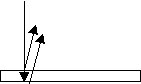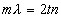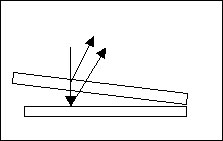Consider a soap film of uniform thickness. If monochromatic light is incident nearly normally on the soap film, light will reflect off the top and bottom of the film and then recombine constructively or destructively. The outcome is determined by two factors: path length difference and the Lloyd's mirror phase change. (Click here to learn about each.) One of the difficulties with this topic that students find challenging is that interference occurs for several thicknesses rather than just one. If the index of refraction of the film is larger than the index on both sides or smaller than both sides, case 1 in the box below applies. If the index of the film in smaller than the index of the material on one side but greater than the index on the otehr side, then the equations are applied as in case 2.

 case 1for m = 0,1,2...yields constructive interferencefor m = 0, 1, 2, ...yields destructive interference n = film index lambda = wavelength of light t = film thickness

 case 2for m = 0,1,2...yields constructive interferencefor m = 0, 1, 2, ...yields destructive interference n = film index lambda = wavelength of light t = film thicknessthin film interference can be applied to good use

go to homework probs - thin film interference

This page was last reviewed by mgosselin 10/09/2005